Click to Chat

1800-1023-196

+91-120-4616500

CART 0

• 0

MY CART (5)

Use Coupon: CART20 and get 20% off on all online Study Material

ITEM
DETAILS
MRP
DISCOUNT
FINAL PRICE
Total Price: Rs.

There are no items in this cart.
Continue Shopping• Complete JEE Main/Advanced Course and Test Series
• OFFERED PRICE: Rs. 15,900
• View Details

Revision Notes on States of Matter

Thus matter is classified mainly into three categories depending upon its physical state namely solid, liquid and gaseous states.Distinction between three states of matter:

S.No

Property

Solid

Liquid

Gas

1

Shape

Definite shape

Indefinite shape

Indefinite shape

2

Volume

Definite Volume

Definite Volume

Indefinite Volume

3

Inter particular Forces

Strong Inter particular Forces

Comparatively weaker  Inter particular Forces

Inte rparticular forces are negligible

4

Inter particular Space

Negligible inter particular space

Comparatively large inter particular space

Very large Inter particular space

5

Particular Motion

Particle motion is restricted to vibratory motion.

Particle motion is very slow

Particle motion is very rapid and also random.

6

Packing of Particles

Particles are very Closely packed

Particles are loosely packed

Particles are very loosely packed

7

Compressibility

Incompressible

Compressible

Highly Compressible

8

Density

Very High Density

Low Density

Very low density

Parameters of Gases

The characteristics of gases are described in terms of following four parameters

Mass

Volume

Pressure

Temperature

1. Mass (m):

The mass of the gas is related to the number of moles as

n = w/M

Where  n = number of moles

w = mass of gas in grams

M = molecular mass of the gas

2. Volume (V):

Since gases occupy the entire space available to them, therefore the gas volume means the volume of the container in which the gas is enclosed.

Units of Volume: Volume is generally expressed in litre (L), cm3 & dm3

1m3 = 103 litre = 103 dm3 = 106 cm3.

3. Pressure:

Pressure of the gas is due to its collisions with walls of its container i.e. the force exerted by the gas per unit area on the walls of the container is equal to its pressure.

Pressure is exerted by a gas due to kinetic energy of its molecules.

As temperature increases, the kinetic energy of molecules increases, which results in increase in pressure of the gas. So, pressure of any gas is directly proportional to its temperature.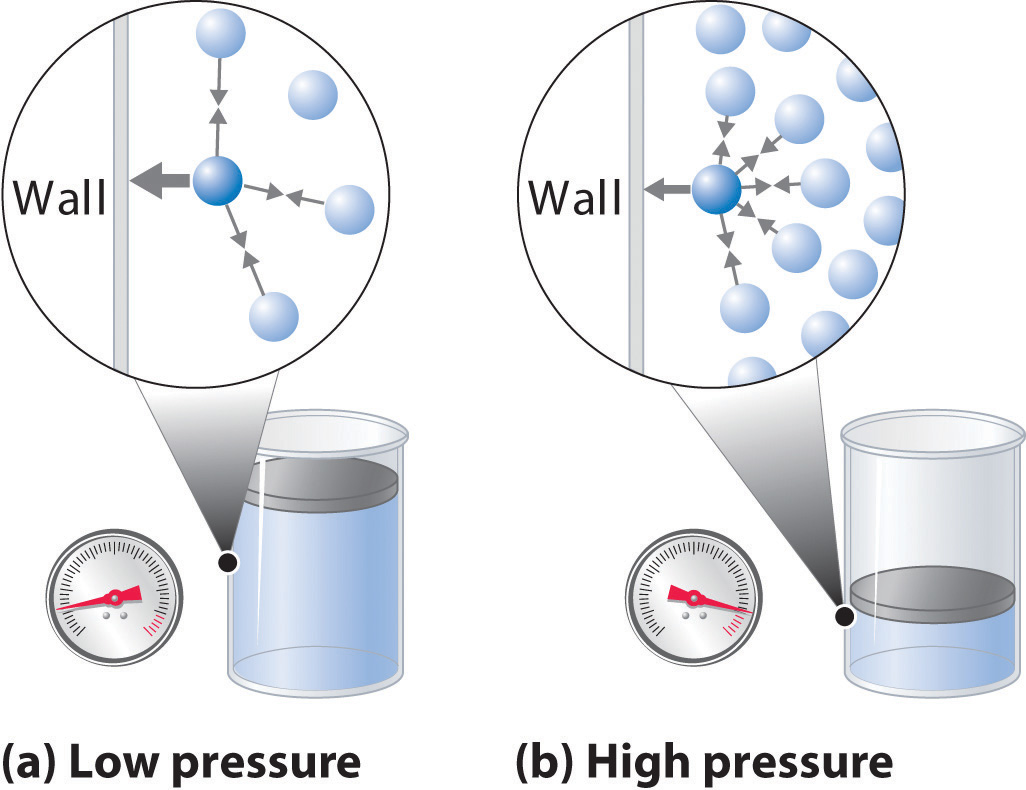Units of Pressure:

The pressure of a gas is expressed in atm, Pa, Nm–2, bar and lb/In2 (psi).

760 mm = 1 atm = 10132.5 KP­a = 101325 Pa = 101325 Nm–2

760 mm of Hg = 1.01325 bar = 1013.25 milli bar = 14.7 lb/2n2 (psi)

3. Temperature (T):

Temperature is defined as the degree of hotness. The SI unit of temperature is Kelvin.  oC and oF are the two other units used for measuring temperature. On the Celsius scale water freezes at 0°C and boils at 100°C where as in the Kelvin scale water freezes at 273 K and boils at 373 K.

K = oC + 273.5

F = (9/5) oC + 32

Gas Laws:

1. Boyle’s Law:-”At constant temperature, the pressure of a fixed amount (i.e., number of moles n) of gas varies inversely with its volume”.

Graphical Representation of Boyle’s Law :

A plot of P versus 1/V at constant temperature for a fixed mass of gas would be a straight line passing through the origin.

A plot of P versus V at constant temperature for a fixed mass of a gas would be a rectangular hyperbola.A plot of P (or V ) versus PV at constant temperature for a fixed mass of a gas is a straight line parallel to the PV axis.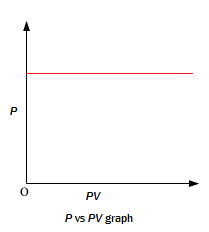2. Charles’ Law:-”At constant pressure, the volume of a given mass of a gas is directly proportional to its absolute temperature”

or

Graphical Representation of Charles’s Law :

1. For a definite mass of the gas a plot of V vs T (oK) at constant pressure is a straight line passing through the origin.2. A plot of V vs t (oC) at constant pressure is a straight line cutting the temperature axis at -273 oC3. Combined Gas Law:-

This law states that “at constant volume, the pressure of a given mass of a gas is directly proportional to its absolute temperature”.

the combination of Boyle’s Law and Charles’ Law:

4. Gay Lussac’s Law:

Where,

P = Pressure of Gas

T= Absolute Temperature

If the pressure and temperature of a gas changes from P1 & T1 to P2 & T2 , volume remaining constant , we have

where,

Pt = Pressure of gas at t oC

Po = Pressure of gas at 0 oC

t = Temperature in oC.

Graphical Representation of Gay-Lussac’s Law“Samples of different gases which contain the same number of molecules (any complexity, size, shape) occupy the same volume at the same temperature and pressure”.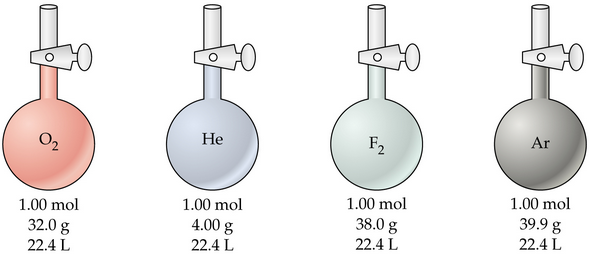It follows from Avogadro’s hypothesis that  (when T and P are constant).

Mathematically

6. Ideal Gas Equation:

Ideal gas obey all the three laws i.e. Boyle’s, Charles’s, and Avogadro‘s law strictly.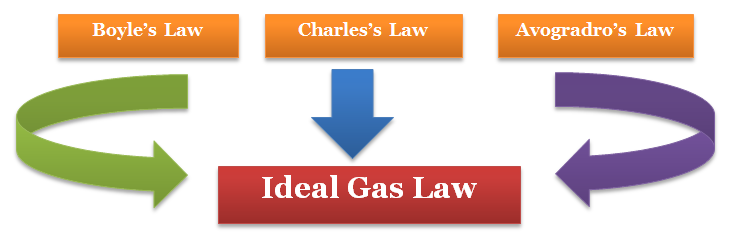pv = nRT

Where,

where R is the constant of proportionality or universal gas constant

The value of R was found out to be

R = 8.314 J mol–1 K–1

R = 0.0821 litre atm K–1 mol–1

R = 2 cal K–1 mol–1

Ideal gas equation is also known as equation ofstate.

7. Dalton’s law of partial pressures:

The total pressure of mixture of non-reactive gases at constant temperature and pressure is equal to    the sum of the individual partial pressures of the gases.

ptotal  = p1 +p2+p3+p4…

p1 = x1 ptotal

p2 = x2 ptotal

p3 = x3 ptotal

Aqueous tension:-

Pressure exerted by saturated water vapour.

pdry gas = pTotal –Aqueous Tension

Gas Eudiometry:

Gas

Absorbing Reagent used:

O3

Turpentine oil

O2

Alkaline pyrogallol

NO

FeSO4 solution

CO2,SO2

Alkali solution (NaOH, KOH, Ca(OH)2, HOCH2CH2NH2, etc.)

NH3

Acid solution or CuSO4 solution

Equation for combustion of hydrocarbons:

CxHy + (x + y/4) O2  ——> xCO2 + y/2  H2O

Kinetic molecular theory of gases:

Gases are made of large number of identical particles (atoms or molecules), which are very small and perfectly hard spheres.

The actual volume of the molecules is negligible as compare to the space between them and hence they are considered as the point  masses.

Interaction between the particles is negligible.

Particles of a gas are always in constant and random motion and the collision between them is perfectly elastic.

The average kinetic energy of the particles of a gas is directly proportional to the absolute temperature.

Pressure of the gas is due to the collision between gas molecules and walls of the container.

The Kinetic Equation

Velocities of gas molecules

Average Velocity

Average velocity =

Root Mean Square Velocity:-

Maxwell proposed the term Urms as the square root of means of square of all such velocities.

also

Most probable velocity:-

It is the velocity which is possessed by maximum no. of molecules.

Furthermore

Kinetic Energy of Gas

As per kinetic equation

For 1 mole m × n = Molecular Mass (M)

Also

Where k is the Boltzmann constant (k = R / N)

Graham’s Law of Diffusion/Effusion:

1. Diffusion: ability of a gas to spread and occupy the whole available volume irrespective of other gases present in the container

2. Effusion: process by which a gas escapes from one chamber of a vessel through a small opening or an orifice

r ∝ 1 / √d
where r is the rate of diffusion and d is the density of the gas.
Now, if there are two gases A and B having r1 and r2 as their rates of diffusion and d1 and d2 their densities respectively. Then

r1 ∝ 1

and

r2 ∝

or

,

The rate of diffusion (r) of a gas at constant temperature is directly preoperational to its pressure

Deviation from ideal gas behavior:

For ideal gas,

Compressibility factor i.e. Z = PV/nRT =1

For non-Ideal gas, Z ≠1

Thus for non-ideal gas,Z can be < 1 or > 1

When Z < 1, it is a negative deviation. It shows that the gas is more compressible than expected from ideal behaviour.

When Z > 1, it is a positive deviation. It shows that the gas is less compressible than expected from ideal behaviour.

1. Causes of deviation from ideal behaviour:

The volume occupied by gas molecules is negligibly small as compared to the volume occupied by the gas.

The forces of attraction between gas molecules are negligible.

2. Van der waals Equation:

Where,a and b are van der waals constants.

At low pressures:

PV = RT – a/V

or

PV < RT

This accounts for the dip in PV vs P isotherm at low pressure

At fairly high pressures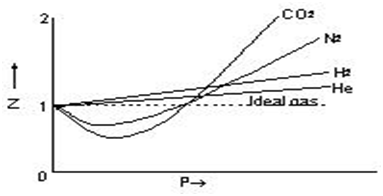a/V2  may be neglected in comparison with P. The Vander Waals equation becomes

PV = RT + Pb

or

PV > RT

This accounts for the rising parts of the PV vs P isotherm at high pressuresBoyle’s Temperature (Tb) :-temperature at which real gas obeys the gas laws over a wide range of pressure.

Tb = a / Rb = 1/2 T1

Liquefaction of gases:

Critical temperature (Tc):- temperature at which a gas liquefies. Tc = 8a / 27Rb

Critical Volume: (Vc) :- volume of one mole of a gas at critical temperature.Vc = 3b

Critical pressure (pc):- pressure of A gas at its critical temperature.  Pc = a/27b2

Molar heat capacity of ideal gases:-the amount of heat required to raise the temperature of 1 mole of a gas trough 10C.

CP -CV = R &

Poisson's ratio (γ) = CP/CV

For monatomic gas Cp = 5 cal and Cv =3 cal

γ = 5/3 = 1.67

For diatomic gas Cp = 7 cal and Cv = 5 cal

γ =7/5 = 1.4

For polyatomic gas Cp = 8 cal and Cv= cal

γ   = 8/6 = 1.33

Also Cp = Cpm,

Where, Cp and Cv are specific heat and m, is molecular weight.

Liquid State:1) Surface Tension (γ):- It is the force acting at right angles to the surface along one centimeter length of the surface.

Surface tension (γ) = Work done / Change in area

Units: CGS: dcm-1

SI:  Nm-1

The surface of the liquid tends to contract to the smallest possible area for a given volume of the liquid i.e. spherical shape.

Surface Tension of liquid decreases with increase of temperature and becomes zero at its critical temperature.

Surface Tension in everyday life:

Cleansing action of soap and detergents.

Efficacy of tooth pastes, mouth washes and nasal jellies.

Viscosity:

It is the force of friction which one part of the liquid offers to another part of the liquid.

Coefficient of viscosity: is the force per unit area required to maintain unit difference of velocity between two parallel layers in the liquid one unit apart.

Units:CGS: dscm-1

S.I:  Nsm-1

Viscosity of liquid decreases with increase in temperature.### Course Features

• 728 Video Lectures
• Revision Notes
• Previous Year Papers
• Mind Map
• Study Planner
• NCERT Solutions
• Discussion Forum
• Test paper with Video Solution